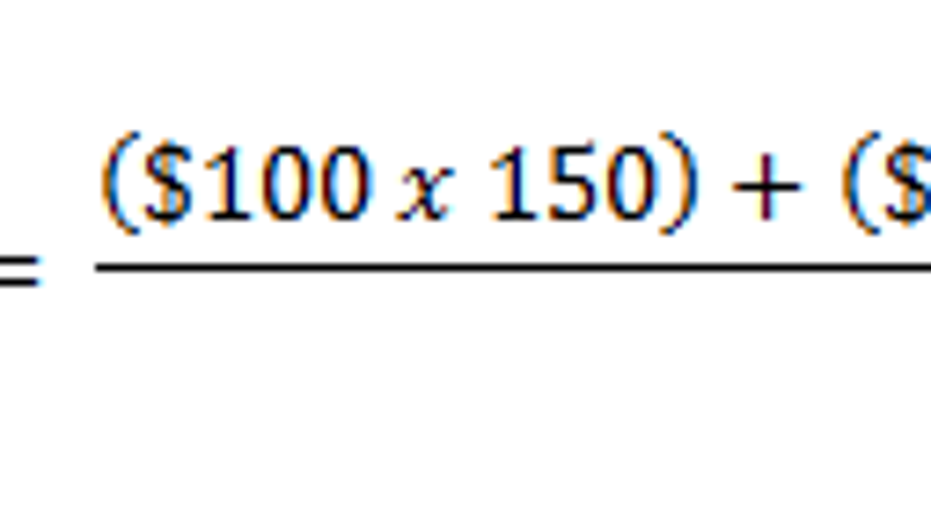# How to Calculate Weighted Average Price per ShareIf you bought all of your stock in a single transaction, it's easy to determine how your investment is performing. Simply look at the current share price and compare it to the price you paid.

However, if you bought your shares in several transactions at different price points, and bought a different number of shares each time, evaluating your investments' performance is a little more complicated. In this case, the best method is to calculate a "weighted average" of the prices you paid.

What is a weighted average?A weighted average is a method of finding the average value of a group of numbers, which takes into account how many times each number occurs, or its importance. A common real-world example is the calculation of a grade-point average in schools, where an "A" carries a greater weight than a "B", which carries a greater weight than a "C", and so on.

How to calculate your weighted average price per shareWhen it comes to buying stock, a weighted average price can be used when shares of the same stock are acquired in multiple transactions over time. This is necessary if the transactions were for different numbers of shares, since the larger purchases contribute more to the average. For example, the mathematical average of \$100 and \$200 is \$150, but if you bought 10 shares of stock at \$100 and only one share at \$200, the lower-priced shares carry more weight when calculating the average price you paid.

In order to calculate your weighted average price per share, you can use the following formula:

In words, this means that you multiply each price you paid by the number of shares you bought at that price. Then, add up all of these results. Finally, divide by the total number of shares you purchased.

This may sound a little complicated, so let's look at an example to illustrate how it works.

An exampleLet's say that you own 500 shares of Microsoft, and you acquired your shares in three separate transactions. You bought the following number of shares at each of the following price points.

• 150 shares at \$100
• 250 shares at \$200
• 100 shares at \$300

In order to calculate your weighted average price per share, simply multiply each purchase price by the amount of shares purchased at that price, add them together, and then divide by the total number of shares. Written as an equation, it looks like this:

Why it's usefulKnowing the weighted average price you paid for each share of stock can help you determine how your investment is performing as a whole, relative to the current share price.